# Algebra - math word problems

1. Borrowed book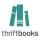Jane must as soon as possible return a borrowed book. She figured that when she read 15 pages a day return book in time. Then she read 18 pages a day and then return the book one day before. How many pages should have a book?
2. Three Titanics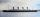Three steamers sailed from the same port on the same day. The first came back on the third day, fourth 4th day and the third returned sixth day. How many days after leaving the steamers met again in the harbor?
3. InfirmaryTwo thirds of children from the infirmary went on a trip seventh went to bathe and 40 children remained in the gym. How many children were treated in the infirmary?
4. Three friends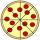Divide 570 euros to three friends so that first will get 50 euros less than the second and third twice more than the first. How many euros will get everyone?
5. DollarsMama split 760 dollars Jane, Dane and Eva as follows: Jane got three times more than Dane and Dane and got 40 more than Eva. How much does get each of them?
6. The book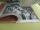If one day you read p pages, you will read the book 24 days. If you read each day 10 pages more, you would read a book in 16 days. How many pages have the book?
7. Shop stores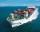Workers untied fruit to stores. In the first quarter shipments, in the second fifth shipment, in the third two-fifths of the rest and in the fourth 231 kilograms. How many fruits were together?
8. Two triangles SSA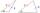Two triangles can be formed with the given information. Use the Law of Sines to solve the triangles. A = 59°, a = 13, b = 14
9. Scouts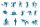Three scouts went on a three day trip. The second day went 4 km more than the first day. The third day went two times less than the first day. They went along 54 km. How many kilometers went every day together?
10. Unknown numberSamuel wrote unknown number. Then he had add 200000 to the number and the result multiply by three. When it calculated he was surprised, because the result would have received anyway, if write digit to the end of original number. Find unknown number.
11. Vacation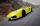Parents piggybacking children on vacation to its grandmother and grandfather at a city distant 150 kilometers. They agreed to meet halfway. Parents will be travel at 90 km/h, grandmother and grandfather at 60 km/h. Parents depart at 12:00 hours. When gran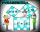Two fifth-graders teams competing in math competitions - in Mathematical Olympiad and Pytagoriade. Of the 33 students competed in at least one of the contest 22 students. Students who competed only in Pytagoriade was twice more than those who just compete
13. Cubes - diffSecond cubes edge is 2 cm longer than the edge of the first cube. Volume difference blocks is 728 cm3. Calculate the sizes of the edges of the two dice.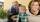Grandson with grandpa they counted how many years have together. Their product is 365. How many years is the sum of their years.
15. Brass tubeThe outer perimeter of brass tube (ρ = 8.5 g/cm3) is 38 cm. Its mass is 5 kg, length 54 cm. What is the pipe wall thickness?
16. Circle sectorCircular sector with a central angle 80 ° has area 1257 cm2. Calculate its radius r.
17. Equilateral cylinderEquilateral cylinder (height = base diameter; h = 2r) has a volume of V = 199 cm3 . Calculate the surface area of the cylinder.
18. Silver medal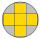To circular silver medal with a diameter of 10 cm is inscribed gold cross, which consists of five equal squares. What is the content area of silver part?
19. Plums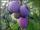In the bag was to total 136 plums. Igor took 3 plums and Mary took 4/7 from the rest. How many plums remained in the bag?
20. Jane and Miro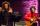Jane brother Miro is 42 years. And he is three times old as it was Jane when Miro was for so many years as there are now Jane. How old is Jane?

Do you have an interesting mathematical word problem that you can't solve it? Submit math problem, and we can try to solve it.

We will send a solution to your e-mail address. Solved examples are also published here. Please enter the e-mail correctly and check whether you don't have a full mailbox.

Please do not submit problems from current active competitions such as Mathematical Olympiad, correspondence seminars etc...